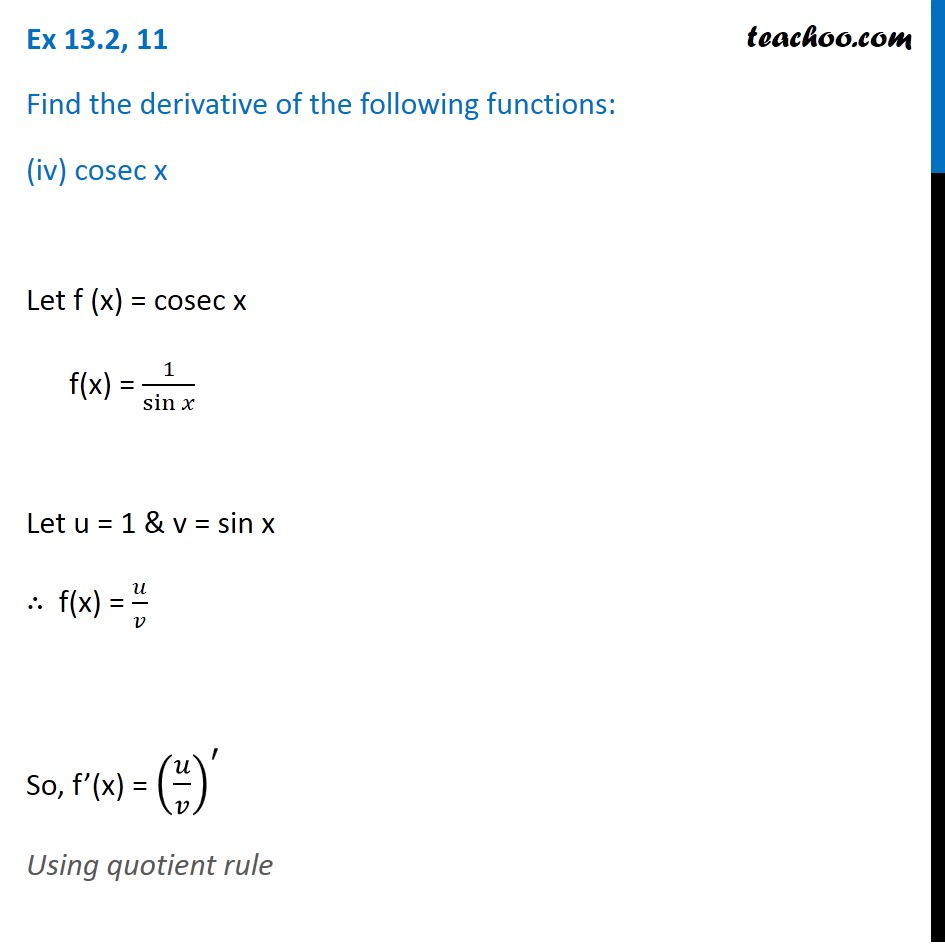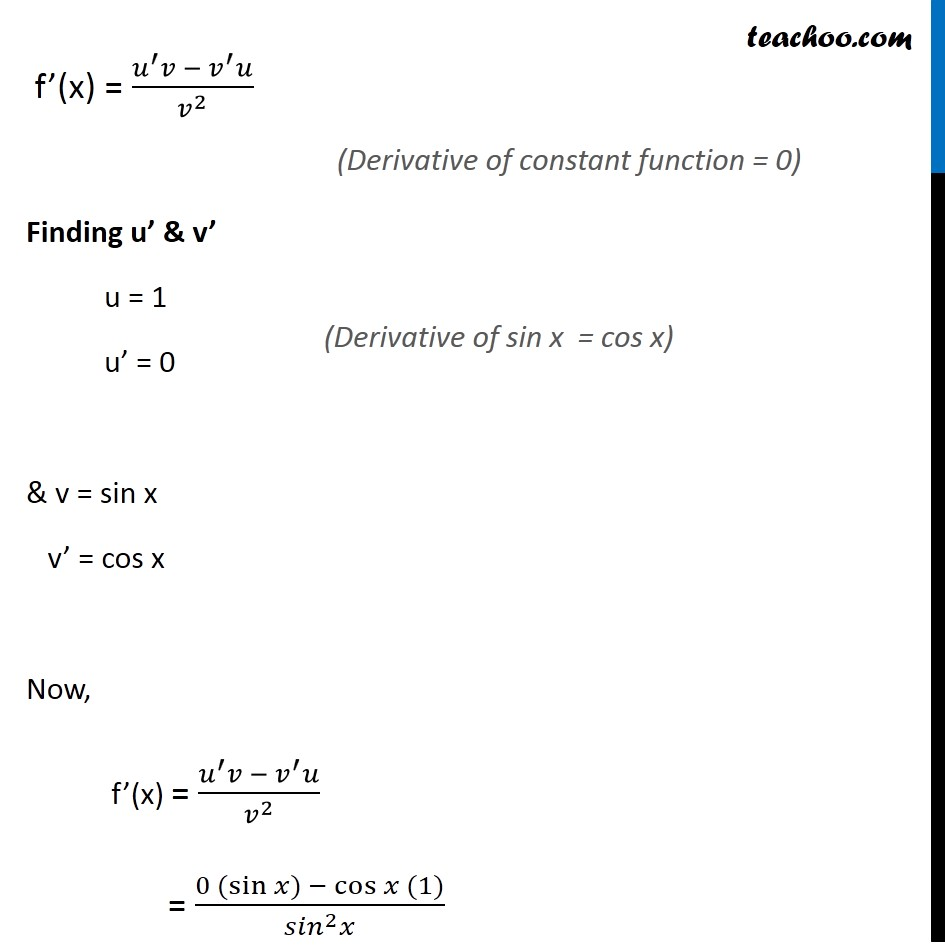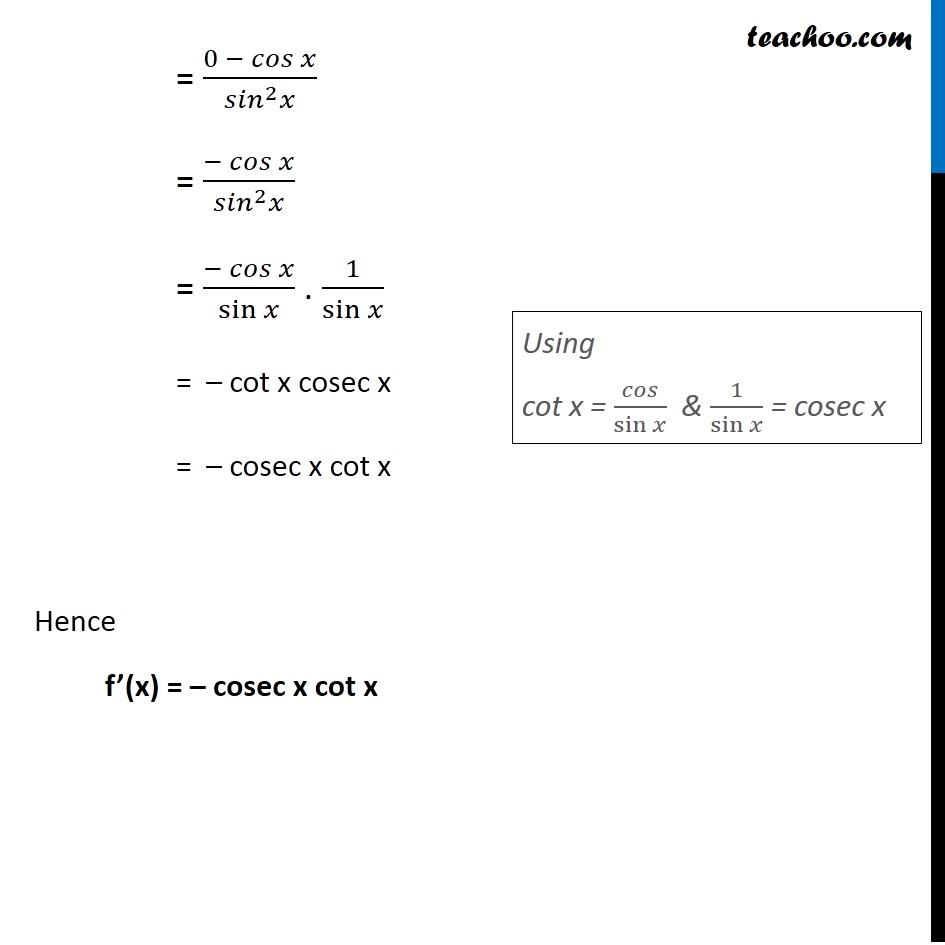Ex 12.2

Chapter 12 Class 11 Limits and Derivatives
Serial order wiseLearn in your speed, with individual attention - Teachoo Maths 1-on-1 Class

### Transcript

Ex 12.2, 11 Find the derivative of the following functions: (iv) cosec x Let f (x) = cosec x f(x) = 1/sin⁡𝑥 Let u = 1 & v = sin x ∴ f(x) = 𝑢/𝑣 So, f’(x) = (𝑢/𝑣)^′ Using quotient rule f’(x) = (𝑢^′ 𝑣 −〖 𝑣〗^′ 𝑢)/𝑣^2 Finding u’ & v’ u = 1 u’ = 0 & v = sin x v’ = cos x Now, f’(x) = (𝑢^′ 𝑣 −〖 𝑣〗^′ 𝑢)/𝑣^2 = (0 (sin⁡〖𝑥) −〖 cos〗⁡〖𝑥 (1)〗 〗)/(〖𝑠𝑖𝑛〗^2 𝑥) (Derivative of constant function = 0) (Derivative of sin x = cos x) = (0 − 𝑐𝑜𝑠 𝑥)/(〖𝑠𝑖𝑛〗^2 𝑥) = (− 𝑐𝑜𝑠 𝑥)/(〖𝑠𝑖𝑛〗^2 𝑥) = (− 𝑐𝑜𝑠 𝑥)/sin⁡𝑥 . 1/sin⁡𝑥 = – cot x cosec x = – cosec x cot x Hence f’(x) = – cosec x cot x Using cot x = 𝑐𝑜𝑠/sin⁡𝑥 & 1/sin⁡𝑥 = cosec x